# Non Verbal Reasoning - Figure Matrix

### Exercise :: Figure Matrix - Section 1

In each of the following questions, find out which of the answer figures (1), (2), (3) and (4) completes the figure matrix ?

11.

Select a suitable figure from the four alternatives that would complete the figure matrix.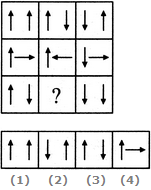A. 1 B. 2 C. 3 D. 4

Explanation:

In each row, the second figure is obtained from the first figure by reversing the direction of the RHS arrow and the third figure is obtained from the second figure by reversing the direction of both the arrows.

12.

Select a suitable figure from the four alternatives that would complete the figure matrix.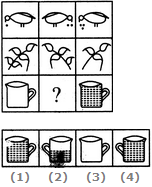A. 1 B. 2 C. 3 D. 4

Explanation:

In each row, the figures are getting laterally inverted in each step. The number of components or the quantities are either increasing or decreasing from left to right sequentially.

13.

Select a suitable figure from the four alternatives that would complete the figure matrix.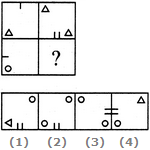A. 1 B. 2 C. 3 D. 4

Explanation:

The second figure is obtained from the first figure by moving the line segment to the opposite side of the square boundary and replacing it with two similar line segments. Also, the element in the lower-left corner gets replaced by two similar elements - one placed in the upper-left and the other placed in the lower-right corner.

14.

Select a suitable figure from the four alternatives that would complete the figure matrix.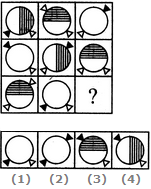A. 1 B. 2 C. 3 D. 4

Explanation:

In each row, there are 3 types of shadings of circles - a circle is unshaded, another circle has its right half shaded with vertical lines and yet another circle has its upper half shaded with horizontal lines. There are three specified positions of the two triangles each of which is used only once in a row. Also, two of the figures in each row have one triangle shaded.

15.

Select a suitable figure from the four alternatives that would complete the figure matrix.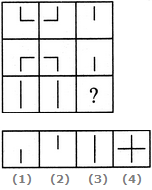A. 1 B. 2 C. 3 D. 4